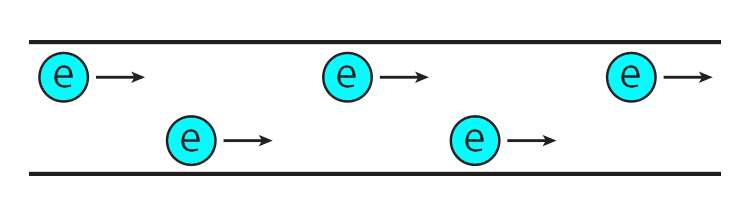# Electrical Current

Current (symbolised by the letter I) is measured in Amps.

One Amp is defined as:

6.24xx10^18 electrons per second passing through a point in a circuit.## Current equals I equals amps

To remember that current is represented by the symbol "I" and is measured in Amps, use the following mnemonic.Currently you're under investigation by the  C.I.A.

 Current = I = A Current = I = Amps